# WitCH 3

First, a quick note about these WitCHes. Any reasonable mathematician looking at such text extracts would immediately see the mathematical flaw(s) and would wonder how such half-baked nonsense could be published. We are aware, however, that for teachers and students, or at least Australian teachers and students, it is not nearly so easy. Since school mathematics is completely immersed in semi-sense, it is difficult to know the rules of the game. It is also perhaps difficult to know how a tentative suggestion might be received on a snarky blog such as this. We’ll just say, though we have little time for don’t-know-as-much-as-they-think textbook writers, we’re very patient with teachers and students who are honestly trying to figure out what’s what.

Now onto WitCH 3, which follows on from WitCH 2, coming from the same chapter of Cambridge’s Specialist Mathematics VCE Units 3 & 4 (2018).* The extract is below, and please post your thoughts in the comments. Also a reminder, WitCH 1 and WitCH 2 are still there, awaiting proper resolution. Enjoy.

* Cambridge is a good target, since they are the most respected of standard Australian school texts. We will, however, be whacking other publishers, and we’re always open to suggestion. Just email if you have a good WitCH candidate, or crap of any kind you wish to be attacked.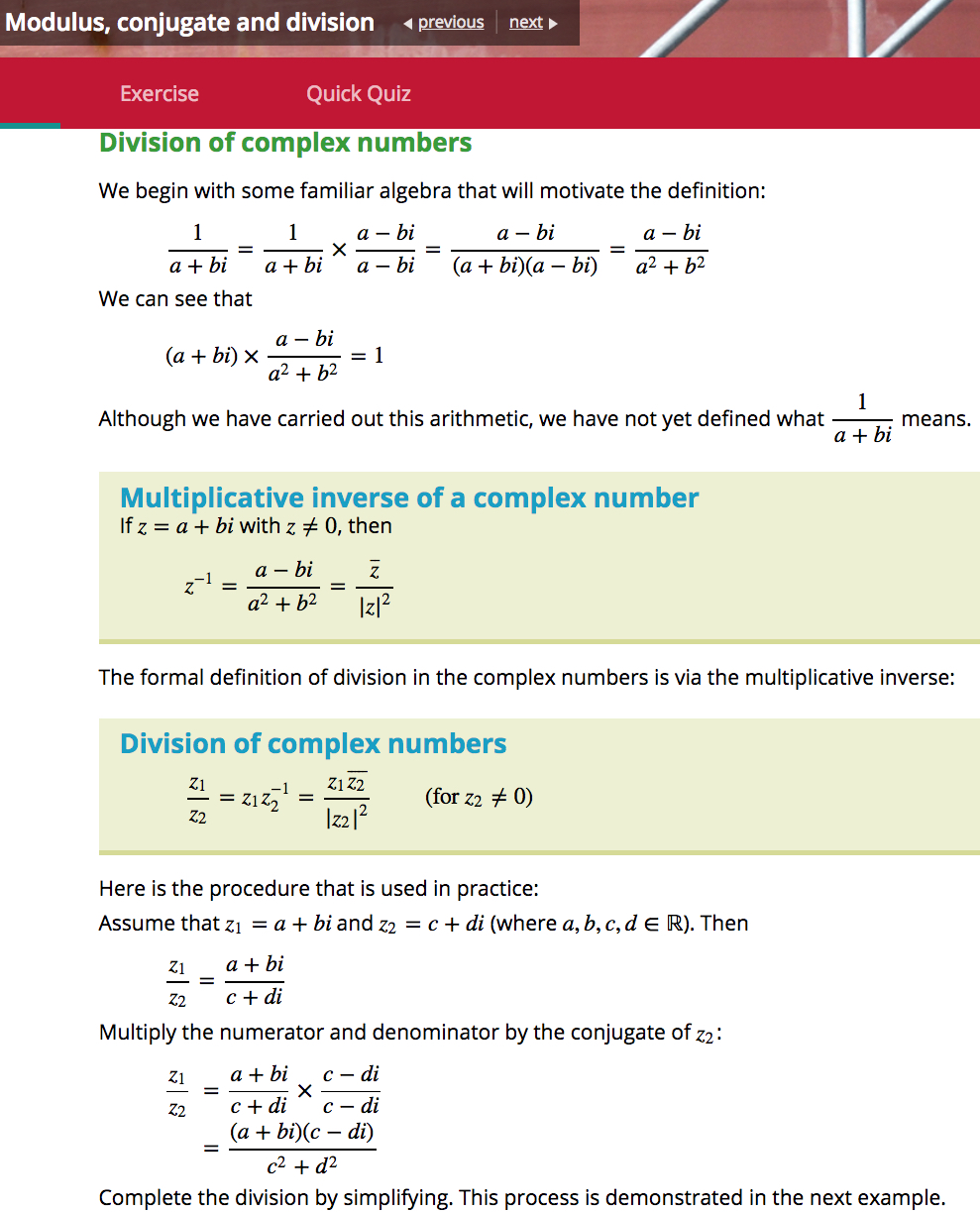## Update (06/02/19)

The above excerpt is indicative of the text’s entire chapter on complex numbers. It is such remarkably poor exposition, the foundations so understated and the direction so aimless, it is almost impossible to find one’s way back to sensible discussion.

Here is a natural framework for a Year 12 topic on complex numbers:

• First, one introduces a new numberfor which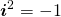.
• One then defines complex numbers, and introduces the fundamental operations of addition and multiplication.
• One then at least states, and hopefully proves, the familiar algebraic properties for complex numbers, i.e. the field laws,and so forth. All these properties are obvious or straight-forward to prove, except for the existence of multiplicative inverses; one has to prove that given any non-zero complex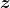there is another complex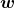with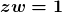.
• That is the basic complex algebra sorted, and then one can tidy up. This includes the definition of division, noting the essential role played by commutativity of multiplication.
• Then, comes the geometry of complex numbers, beginning with the definition and algebraic properties of the conjugate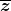and modulus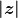,  the interpretation of these quantities in terms of the complex plane, and polar form.
• Finally, the algebra and geometry of complex numbers are related: the parallelogram interpretation of addition, the trigonometric-polar interpretation of multiplication, roots of complex numbers and so forth.

Must complex numbers be taught in this manner and in this order? No and yes. One obvious variation is to include a formal definition of a complex number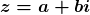as an ordered pair; as Damo remarks below, this is done as an asterisked section in Fitzpatrick and Galbraith. Though unnervingly abstract, the formal definition has the non-trivial advantage of reinforcing, almost demanding, the interpretation of complex numbers as points in the complex plane. More generally, one can emphasise more or less of the theoretical underpinnings and, to an extent, change the ordering.

But, one can only change the ordering and discard the theory so much, and no more. Complex numbers are new algebraic objects, and defining and clarifying the algebra is critical, and this fundamentally precedes the geometry.

What is the Cambridge order? The text starts off well, introducingwith,  and then immediately goes off the rails by declaring that. Then, in brief, the text includes:

(a) an invalid treatment of the square roots of negative numbers;

(b) complex addition stated, presumably defined, with the inverse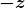introduced but not named;

(c) complex subtraction, followed by an almost invisible statement of the relevant field laws, none of which are proved or assigned as exercises;

(d) scalar multiplication;

(d) the complex plane and “the representation of the basic operations on complex numbers”;

(e) complex multiplication defined, with an almost invisible statement of field laws, none of which are proved or assigned as exercises, and with no mention of the question of multiplicative inverses;

(f) the geometry of multiplication by;

(g) the modulus of a complex number defined, with algebraic properties (including) stated and assigned as exercises;

(h) the conjugate of a complex number defined, with algebraic properties stated and either proved or assigned as exercises.

(h) Finally, as excerpted above, it’s on to reciprocals of complex numbers, multiplicative inverses in terms of modulus and conjugate, and division.

(i) This is followed by sections on polar form, de Moivre’s theorem and so forth;

(j) CAS garbage is, of course, interspersed throughout. (Which is not all Cambridge’s fault, but the text is no less ugly for that.)

At no stage in the text’s exposition is there any visible concern for emphasising or clarifying foundations, or for following a natural mathematical progression. There is too seldom an indication of what is being defined or assumed or proved.

What is the point? Yes, one can easily be overly theoretical on this topic, but this is Year 12 Specialist Mathematics. It is supposed to be special. The students have already been introduced to complex numbers in Year 11 Specialist. Indeed, much of the complex material in the Year 11 Cambridge text is repeated verbatim in the Year 12 text. Why bother? The students have already been exposed to the nuts and bolts, so why not approach the subject with some mathematical integrity, rather than just cutting and pasting aimless, half-baked nonsense?

Now, finally and briefly, some specific comments on the specific nonsense excerpted above.

• division of complex numbers has already appeared in the text, in the list of (unproved) properties of the modulus.
• the algebraic manipulation of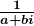is unfamiliar and unmotivated and, as is admitted way too late, is undefined. There is a place for such “let’s see” calculations – what mathematicians refer to as formal calculations –  but they have to be framed and be motivated much more carefully.
• There is no need here for a “let’s see” calculation. The critical and simple observation is thatis real. It is then a short step to realise and to prove that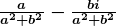acts as, and thus is, the multiplicative inverse of.
• Having finally admitted thathas not been defined, the text goes on to not define it again. The text states the multiplicative inverse of, but it is not clear whether this statement amounts to a definition or a conclusion.
• Division of complex numbers is then defined with needless subscripts and, more importantly, with no mention of the fundamental role of commutativity of multiplication.
• Throughout, the use of conjugate and modulus is muddying rather than clarifying.
• At no stage is it made clear whymakes sense in contrast to, for example, the non-sense of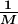for a matrix.

## 7 Replies to “WitCH 3”

1.Number 8 says:

Oh how I dislike this… just like dividing by a surd (probably something else you could pick on if you had more time) there is a difference between doing the actual division, creating a fraction and rationalising (or in this case realising – sarcasm intended) the denominator.

A real (or rational sometimes) denominator might look nice, but I see no reason why fractions have to be given in this form for the division process to be complete.

But then I’m not even trying to pretend to be a half-reasonable mathematician.

2.Damo says:

To begin with, is the ‘familiar algebra’ really that familiar? Maybe to teachers who are already, well, familiar with the topic, but for the average student coming across division of complex numbers for the first time, I would guess that this algebra is neither familiar nor obvious. Also, I like that this algebra is motivating the definition. I have a picture in my head, where the algebra is saying “there’s a good definition, you’re doing a bang up job. Sure lots of students are confused by you at first, but give it time and soon enough they’ll understand that division of complex numbers means, um, multiplying by the conjugate of the denominator and then dividing by the square of the modulus of the denominator. I think I’ve got that right? That is what you’re saying? Cool. Stay awesome.”

1.marty says:

Hi Damo. Good question, as to whether the algebra is in any sense “familiar”. Whether or not the algebra is familiar, it is meaningless.

1.Damo says:

Indeed. This whole page falls under the “it shouldn’t be that hard” category. As far as defining division, all it really requires is something along the lines: If z1*z2=z3 then z3/z2=z1. The only reason I can think of for including the multiplicative inverse is if you wanted to show that the set of complex numbers is a field. Something that Fitzgerald and Galbraith did in Pure Mathematics, back in the day. There is a place for the bottom section, the “procedure in practice” although you would want accompanying examples.

1.marty says:

Damo, I think you’re being led by the awfulness of the Cambridge disease to the wrong cure. Indeed the page doesn’t have to be that hard, and F & G isn’t that hard. But what is the text trying to accomplish on this page? What *should* one try to accomplish? What is the point?

3.Damo says:

OK. I’m finding this more difficult to nail down then I anticipated. I’ll give it my best, but go easy!
Through the first part of this section they are trying to define division by way of the multiplicative inverse. However, their definition of the multiplicative inverse is dodgy.

So what have they actually done? They took a complex number 1/(a+bi) and ‘realised’ the denominator. Then, by way of a result which presents as an ‘aside’, they note “we can see” that (a+bi) * this new number = 1. However, I would wager that this is not obvious to your average student. Also, this property is central to the idea of a multiplicative inverse and, as such, should be where they start (as outlined below). They then define this new number as the multiplicative inverse of a+bi. But this is trickery. Rather than defining anything, all they have really done is (apologies for the following notation) shown that the reciprocal of a complex number z=a+bi after you have realised the denominator is (a-bi)*(a^2+b^2)=conj(z)/(mod(z))^2 (with a side note that if you multiply this by z you get 1).

If you aree going to define division using the multiplicative inverse, then you should start by defining the multiplicative inverse properly. That is, the multiplicative inverse is z^-1 so that z*z^-1=1. With complex numbers, we can then find the multiplicative inverse of z=x+yi in the form z^-1=a+bi by letting z*z^-1=(x+yi)*(a+bi)=1+0i. Using familiar algebra, we can expand, equate the real and imaginary parts and solve those two equations to get a=x/(x^2+y^2) and b=-y/(x^2+y^2).

They then go on to define division as being z1/z2=z1*z2^-1=z1*conj(z2)/(mod(z2))^2. However, this would have been enough: z1/z2=z1*z2^-1.

Hopefully some of what I’ve said is near the mark. I haven’t really touched on what we should be trying to achieve. Maybe after dinner!

1.marty says:

Damo, that was an excellent go at unravelling what the text is tepidly attempting. I’ll update the post in the next day or so.

This site uses Akismet to reduce spam. Learn how your comment data is processed.